# SBI Clerk Mains Quantitative Aptitude (Day-06)

Dear Aspirants, Our IBPS Guide team is providing new series of Quantitative Aptitude Questions for SBI Clerk Mains 2020 so the aspirants can practice it on a daily basis. These questions are framed by our skilled experts after understanding your needs thoroughly. Aspirants can practice these new series questions daily to familiarize with the exact exam pattern and make your preparation effective.

Start Quiz

Ensure Your Ability Before the Exam – Take SBI Clerk 2020 Mains Free Mock Test

Data Interpretation

Directions (1 – 5): Study the following information carefully and answer the given questions?

The following table shows the amount invested by 5 different persons, the number of years and the rate of interest and the total amount received using simple interest. Some values are missing here.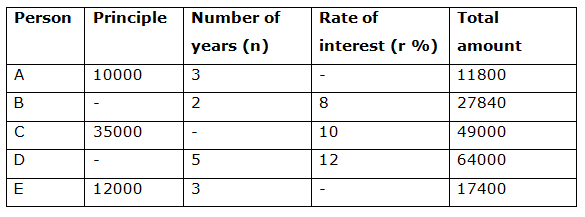1) Find the difference between the amount invested by the person B to that of person D?

a) Rs. 20000

b) Rs. 24000

c) Rs. 16000

d) Rs. 18000

e) None of these

2) Find the ratio between the rate of interest of person A to that of person C?

a) 3: 5

b) 11: 17

c) 23: 15

d) 5: 9

e) None of these

3) Find the average amount received by all the given persons together?

a) Rs. 35612

b) Rs. 33985

c) Rs. 35936

d) Rs. 34008

e) None of these

4) Find the number of years the amount invested by person C?

a) 3 years

b) 4 years

c) 5 years

d) 2 years

e) None of these

5) Find the difference between the average rate of interest for person A, B and C together to that of the person A, D and E together?

a) 8 %

b) 5 %

c) 3 %

d) 6 %

e) None of these

Number series

Directions (6 – 10): The questions below are based on the given Series-I. The series-I satisfy a certain pattern, follow the same pattern in Series-II and answer the questions given below.

6)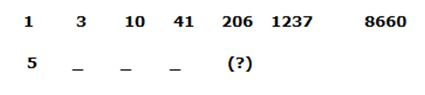a) 460

b) 406

c) 686

d) 866

e) 506

7)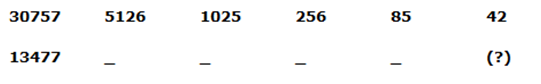a) 24

b) 28

c) 32

d) 18

e) 48

8)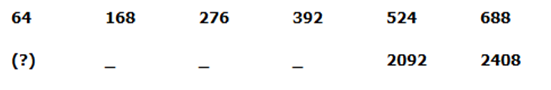a) 961

b) 1024

c) 512

d) 125

e) 343

9)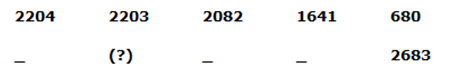a) 4207

b) 4205

c) 4208

d) 4209

e) 4206

10)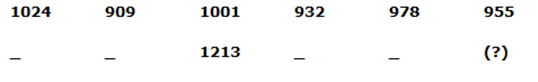a) 1144

b) 1121

c) 1236

d) 1190

e) 1167

Directions (1-5) :

SI = PNR/100

SI = Total amount – Principle

The amount invested by the person B

= > 27840 – P = P*2*8/100

= > 2784000 – 100P = 16P

= > 2784000 = 116P

= > Principle amount (B) = 2784000/116 = Rs. 24000

The amount invested by the person D

= > 64000 – P = P*5*12/100

= > 6400000 – 100P = 60P

= > 6400000 = 160P

= > Principle amount (D) = 6400000/160 = Rs. 40000

Required difference = 40000 – 24000 = Rs. 16000

The rate of interest of person A

= > 1800 = (10000*3*r1)/100

= > 180000/30000 = r1

= > r1 = 6 %

The rate of interest of person C = 10 %

Required ratio = 6: 10 = 3: 5

The average amount received by all the given persons together

= > (11800 + 27840 + 49000 + 64000 + 17400)/5

= > 170040/5 = Rs. 34008

The number of years the amount invested by person C

= > (49000 – 35000) = 35000*n*10/100

= > 14000*100/(350000) = n

= > n = 4 years

The rate of interest of person A

= > 1800 = (10000*3*r1)/100

= > 180000/30000 = r1

= > r1 = 6 %

The average rate of interest for person A, B and C together

= > (6 + 8 + 10)/3 = 24/3 = 8 %

The rate of interest of person E

= > 5400 = (12000*3*r2)/100

= > 540000/36000 = r2

= > r2 = 15 %

The average rate of interest for person A, D and E together

= > (6 + 12 + 15)/3 = 33/3 = 11 %

Required difference = 11 – 8 = 3 %

Directions (6 – 10):

Series I pattern:

1 * 2 + 1 = 3

3 * 3 + 1 = 10

10 * 4 + 1 = 41

41 * 5 + 1 = 206

206 * 6 + 1 = 1237

1237 * 7 + 1 = 8660

Series II pattern:

5 * 2 + 1 = 11

11 * 3 + 1 = 34

34 * 4 + 1 = 137

137 * 5 + 1 = 686

686 * 6 + 1 = 4117

4117 * 7 + 1 = 28820

Series I pattern:

30757 – 1 ÷ 6 = 5126

5126 – 1 ÷ 5 = 1025

1025 – 1 ÷ 4 = 256

256 – 1 ÷ 3 = 85

85 – 1 ÷ 2 = 42

Series II pattern:

13477 – 1 ÷ 6 = 2246

2246 – 1 ÷ 5 = 449

449 – 1 ÷ 4 = 112

112– 1 ÷ 3 = 37

37 – 1 ÷ 2 = 18

Series I pattern:Series II pattern:Series I pattern:

2204 – 12 = 2203

2203 – 112 = 2082

2082 – 212 = 1641

1641 – 312 = 680

Series II pattern:

4207 – 12 = 4206

4206 – 112 = 4085

4085 – 212 = 3644

3644 – 312 = 2683

Series I pattern:Series II pattern: